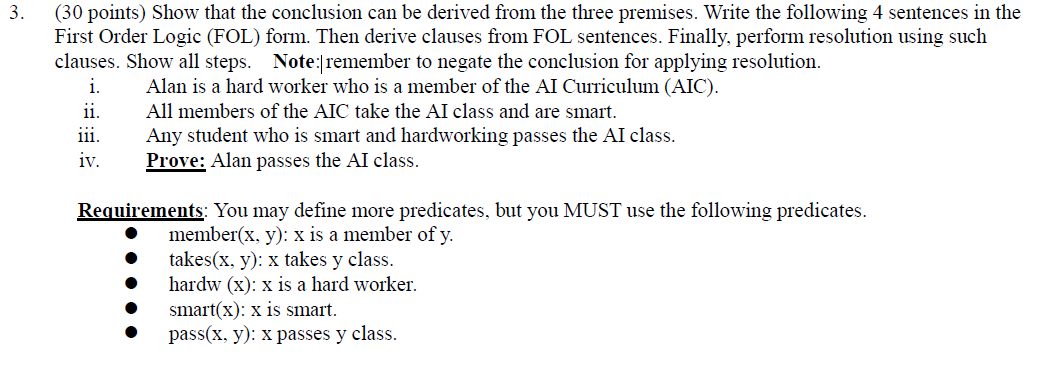Home / Expert Answers / Computer Science / 30-points-show-that-the-conclusion-can-be-derived-from-the-three-premises-write-the-following-4-pa713

# (Solved): (30 points) Show that the conclusion can be derived from the three premises. Write the following 4 ...(30 points) Show that the conclusion can be derived from the three premises. Write the following 4 sentences in the First Order Logic (FOL) form. Then derive clauses from FOL sentences. Finally, perform resolution using such clauses. Show all steps. Note:|remember to negate the conclusion for applying resolution. i. Alan is a hard worker who is a member of the AI Curriculum (AIC). ii. All members of the AIC take the AI class and are smart. iii. Any student who is smart and hardworking passes the AI class. iv. Prove: Alan passes the AI class. Requirements: You may define more predicates, but you MUST use the following predicates. - member $$(x, y): x$$ is a member of $$y$$. - takes(x, y): $$x$$ takes y class. - hardw (x): $$\mathrm{x}$$ is a hard worker. - $$\operatorname{smart}(\mathrm{x}): \mathrm{x}$$ is smart. - $$\operatorname{pass}(\mathrm{x}, \mathrm{y}): \mathrm{x}$$ passes y class.

We have an Answer from Expert